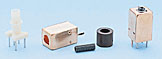Slug Tuned Inductor

This side presents some facts about slug tuned inductors
I have been measure the inductance for different winding turns.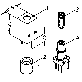All contribution to this page are most welcome

Fabr Neosid typ 7x1S
The 7V1S type which I have been testing has a frequency range from 50-200MHz.
The AL value for this coil is 4,5 nH/n2
The color of the slug is Green.

Formula : L (nH) = N2 *AL

Where:
L = inductance in nanohenrys
n = number of turns
AL = inductance factorThe diagram at the right shows the inductance relative to number of turns.
There is 4 curves:

1. The slug is at the bottom of the can.
2. The top of the slug is in line with the top of the can.
3. The slug is totally removed.
4. A theoretically calculated curve.

Klick on the diagram to se a larger pic.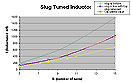The diagram at the right is the same diagram as above but has different scale for the lower inductance.

Conclution: As you can see from the diagram the theoretical value match the blue curve very well.
The area between the Green and the Yellow curve is the span in wich you can adjust you inductance value.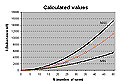The diagram at the right shows the calculated values from MIN, MAX inductance.

Where can I get this can?
You can order this can from ELFA in Sweden article number 58-610-59.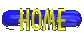[ About me | Acronyms  | CW | Data Sheets | Docs | Download | E-mail | HOME | Ham projects | Hobby circuits | Photo galery | PIC | QTH photos |